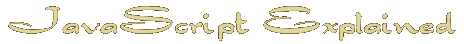Start | Time | IF | Date Object | Arrays | Avoid the work | Hide email | Random | Intigrate HTML | TestThe " IF " statement

So now we can mess around with years and keep our webpage current without having to change it every January but sometimes using a variable can upset the text.
We can easily print "I have now had a computer for () years." but what if the answer is only one.
"I have now had a computer for 1 years." is pretty bad grammar so we need to be able to control 'years' and make it 'year' if the answer is 1.

Introducing the 'if' statement.
Remember 'age' is the variable that may come out as 1.
We need another variable that we will call 'years'.
There are some rules about what can go in the 'if' statement but as an introduction I'll keep it simple.
First we assign our new variable with its normal content
then if age is 1 we change the content.
The double = sign is common to most computer languages when used in the if statement.
Other comparisons are :
< less than
> greater than
<= less than or equal
>= greater than or equal
!= not equal
 years = 'years' ; if ( age == 1 ) years = 'year' ;
Pretty simple right?

Now all we have to do is include our new variable 'years' in the document.write( )
Here is the whole thing.

One thing to notice here is that I needed a space between age and years
so I had to add + ' ' +.
The space is actually in normal text.

Another thing you will see is that I have now begun to use ; at the end of statements.
It changes nothing but can be used to group statements on one line.

For instance the first 4 lines of this script could be written
now = new Date( ); yr = now.getFullYear( ); age = yr - 2003 ; years = 'years' ;

Although it is optional in JavaScript in other computer languages it is necessary so I normally put it in there.

Now I will show you how to change multiple things within an 'if' statement.
It is done by using curly brackets like this
 if (h > 12 ) { timeofday='Afternoon' ; noon = 'pm' ; }

Also you can have more than one option in the if statement separated by && (and ) or || (or)
 if (noon == 'pm' && hr < 6 ) // If it is pm and before 6 on the 12 hour clock document.write ( 'It is Afternoon' ) if ( h > 23 || h< 6 ) // If it is after 11 pm or before 6 am document.write ( 'It is night time' )

Before we move on there is one more thing you will often see in programming and that is explanatory remarks or comments.
Sometimes these can make a script much longer but they make things easier to find.
If you use // at the beginning of a line that line will be ignored by JavaScript.
It is the equivalent of using <! in HTML
Here is what our script could look like if I put in some explanations.

Obviously I went overboard with this but the occasional explanation can make editing much easier.

Next : More about time

Home